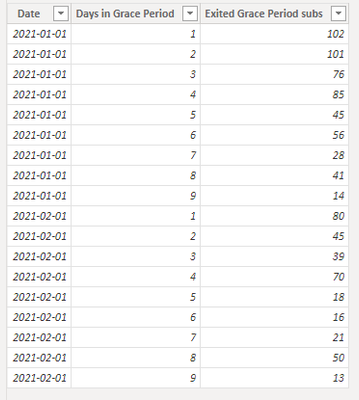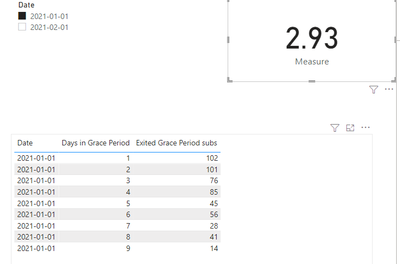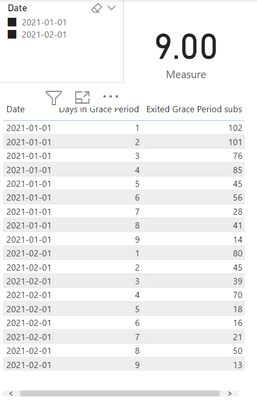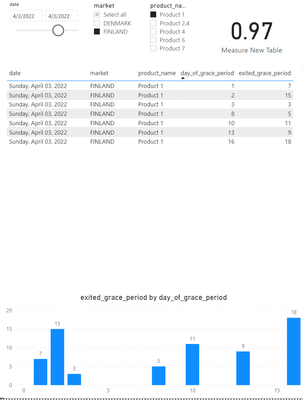cancel
Showing results for
Did you mean:

Fabric is Generally Available. Browse Fabric Presentations. Work towards your Fabric certification with the Cloud Skills Challenge.Regular Visitor

## Getting number of days it took to reach target

Hello!

I am trying to calculate how many days it took to reach a target based on historical data.

Data:

 Days in Grace Period Exited Grace Period subs 1 102 2 101 3 76 4 85 5 45 6 56 7 28 8 41 9 14

I am trying to calculate how many days 50% of all subs spent in Grace Period.

I think it should look something like this:
Total_subs = 102 + 101 + 76 + 85 + 45 + 56 + 28 + 41 + 14 = 548

50% Target = 548 / 2 = 274

So how many days does it takes that first 50% (274) subs to exit Grace Period?
Should it be here some kind of loop?

day_count = 0
1. if 274 - 102 >=  102 then day_count = day_count + 1 else day_count = day_count + (274 / 102 )
2. if 172 - 101 >= 101 then day_count= day_count + 1 else day_count = day_count + (172 / 101)
3. if 71 - 76 >= 76 then day_count = day_count + 1 else day_count = day_count + (71 / 76)
return day_count

And as the result, I can see the total number of days as 2.93 for this example

I hope it makes sense.

6 REPLIES 6Resident Rockstar

How large of a data set are you going to be working with?  I've figured out a very complex way of expanding your table to the Total Number of Exited Subs, then doing math to get to the 50% mark, but it takes A LOT of overhead.  If you table is small like the sample, should be OK.  If you mocked up something small and we are dealing with hundreds of thousands of actual Exited Subs, it wont work...?

Please give Kudos or Mark as a Solution!

Proud to give back to the community!
Thank You!Regular Visitor

There are thousands of records.
Initial data look like this (data for every day for the last 3 years). The dates I'd like to use as a slicer.

 Date Days in Grace Period Exited Grace Period subs 01/01/2021 1 102 01/01/2021 2 101 01/01/2021 3 76 01/01/2021 4 85 01/01/2021 5 45 01/01/2021 6 56Community Support

Hi @Philipp_L ,

My Smaple:Try this code.

``````Measure =
VAR _Total_Subs = CALCULATE(SUM('Table'[Exited Grace Period subs]),ALLEXCEPT('Table','Table'[Date]))
VAR _HALF_OF_TOTAL= DIVIDE( _Total_Subs ,2)
VAR _VIRTUAL_TABLE = ADDCOLUMNS(ALL('Table'),"RunningTotal",CALCULATE(SUM('Table'[Exited Grace Period subs]),FILTER(ALLEXCEPT('Table','Table'[Date]),'Table'[Days in Grace Period]<=EARLIER([Days in Grace Period]))))
VAR _MAXDAY = MAXX(FILTER(_VIRTUAL_TABLE,[Date] = MAX('Table'[Date])&&[RunningTotal]<=_HALF_OF_TOTAL),[Days in Grace Period])
VAR _MAXSUM = MAXX(FILTER(_VIRTUAL_TABLE,[Date] = MAX('Table'[Date])&&[RunningTotal]<=_HALF_OF_TOTAL),[RunningTotal])
VAR _SUB_NEXTDAY = SUMX(FILTER(_VIRTUAL_TABLE,[Date] = MAX('Table'[Date])&&[Days in Grace Period] = _MAXDAY+1),[Exited Grace Period subs])
RETURN
IF(_HALF_OF_TOTAL = _MAXSUM, _MAXDAY, _MAXDAY+DIVIDE(_HALF_OF_TOTAL - _MAXSUM ,_SUB_NEXTDAY))``````

Result is as below.Best Regards,
Rico Zhou

If this post helps, then please consider Accept it as the solution to help the other members find it more quickly.Regular Visitor

Hi @v-rzhou-msft

This solution is very close to what I'm looking for, but there are two issues:
1. It only measures within a single day, not a range of days.
2. There is no way to filter by other dimensions. In the table, I have country and product information. Sorry for not mentioning it earlier.

Could you please let me know if it is possible to measure by a range of the dates?Community Support

Hi @Philipp_L ,

1. It only measures within a single day, not a range of days.

I think you can change the slicer from list to range to achieve your goal.

2. There is no way to filter by other dimensions. In the table, I have country and product information. Sorry for not mentioning it earlier.

``````Measure =
VAR _Total_Subs = CALCULATE(SUM('Table'[Exited Grace Period subs]),ALLEXCEPT('Table','Table'[Date],'Table'[Country])...)
VAR _HALF_OF_TOTAL= DIVIDE( _Total_Subs ,2)
VAR _VIRTUAL_TABLE = ADDCOLUMNS(ALL('Table'),"RunningTotal",CALCULATE(SUM('Table'[Exited Grace Period subs]),FILTER(ALLEXCEPT('Table','Table'[Date],'Table'[Country]...),'Table'[Days in Grace Period]<=EARLIER([Days in Grace Period]))))
VAR _MAXDAY = MAXX(FILTER(_VIRTUAL_TABLE,[Date] = MAX('Table'[Date])&&[RunningTotal]<=_HALF_OF_TOTAL),[Days in Grace Period])
VAR _MAXSUM = MAXX(FILTER(_VIRTUAL_TABLE,[Date] = MAX('Table'[Date])&&[RunningTotal]<=_HALF_OF_TOTAL),[RunningTotal])
VAR _SUB_NEXTDAY = SUMX(FILTER(_VIRTUAL_TABLE,[Date] = MAX('Table'[Date])&&[Days in Grace Period] = _MAXDAY+1),[Exited Grace Period subs])
RETURN
IF(_HALF_OF_TOTAL = _MAXSUM, _MAXDAY, _MAXDAY+DIVIDE(_HALF_OF_TOTAL - _MAXSUM ,_SUB_NEXTDAY))``````

Best Regards,
Rico Zhou

If this post helps, then please consider Accept it as the solution to help the other members find it more quickly.Regular Visitor

1. It only measures within a single day, not a range of days.

I think you can change the slicer from list to range to achieve your goal.

Yeah, I tried even with the initial data, but it doesn't work. For single days the measure equals 2.93 and 3.17 but with the range, it is 9, which doesn't make sense.2. There is no way to filter by other dimensions. In the table, I have country and product information. Sorry for not mentioning it earlier.

I have added as you suggested but it doesn't seem to work properly even for a single day 🤔Here is the link to the updated power bi file:Announcements#### Power BI Monthly Update - November 2023

Check out the November 2023 Power BI update to learn about new features.#### The largest Power BI and Fabric virtual conference

130+ sessions, 130+ speakers, Product managers, MVPs, and experts. All about Power BI and Fabric. Attend online or watch the recordings.Top Solution Authors
Top Kudoed Authors
Users online (6,092)